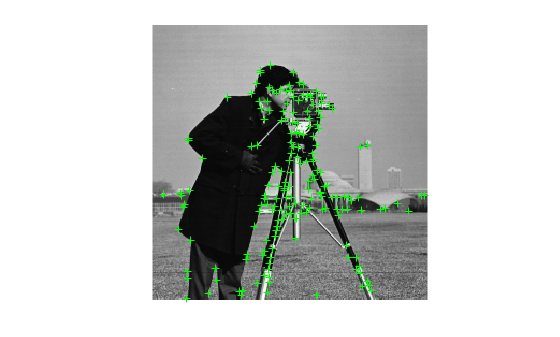# plot

## Syntax

``plot(points)``
``plot(points,ax)``
``plot(points,ax,Name=Value)``

## Description

example

````plot(points)` plots points in the current axis.```
````plot(points,ax)` plots points in the specified axis.```
````plot(points,ax,Name=Value)` specifies options using one or more name-value arguments in addition to any combination of arguments from previous syntaxes. For example, `plot(ShowOrientation=true)` renders the orientation in the display plot.```

## Examples

collapse all

`I = imread("cameraman.tif");`

Detect KAZE points from the image.

`points = detectKAZEFeatures(I);`

Extract KAZE features from the detected points.

`[features,valid_points] = extractFeatures(I,points);`

Plot the 10 strongest points and show their orientations.

```imshow(I) hold on strongestPoints = selectStrongest(valid_points,10); plot(strongestPoints,showOrientation=true) hold off``````I = imread("cameraman.tif"); points = detectSURFFeatures(I); [features, valid_points] = extractFeatures(I,points);```

Visualize 10 strongest SURF features, including their scales and orientation which were determined during the descriptor extraction process.

```imshow(I); hold on; strongestPoints = valid_points.selectStrongest(10); strongestPoints.plot("showOrientation",true);````I = imread("cameraman.tif");`

Detect corner features.

`featurePoints = detectHarrisFeatures(I);`

Plot feature image with detected features.

```imshow(I); hold on; plot(featurePoints);```## Input Arguments

collapse all

Points object,specified as an M-by-2 matrix of M number of [x y] coordinates, or as one of the point feature objects described in Point Feature Types. The object contains information about the feature points detected in the input image. To obtain points, use the appropriate detect function that pairs with the point feature type.

Handle to use for display. You can set the handle using `gca`.

### Name-Value Arguments

Specify optional pairs of arguments as `Name1=Value1,...,NameN=ValueN`, where `Name` is the argument name and `Value` is the corresponding value. Name-value arguments must appear after other arguments, but the order of the pairs does not matter.

Before R2021a, use commas to separate each name and value, and enclose `Name` in quotes.

Example: `plot(ShowOrientation=true)` renders the orientation in the display plot.

Display scaled circle, specified as `true` or `false`. When you set this value to `true`, the object draws a circle proportional to the scale of the detected feature, with the feature point located at its center. When you set this value to `false`, the object turns the display of the circle off.

The algorithm represents the scale of the feature with a circle of `6`*`Scale` radius. The algorithm uses this equivalent size of circular area to compute the orientation of the feature.

Display feature point orientation, specified as `true` or `false`. When you set this value to `true`, the object draws a line corresponding to the point's orientation. The object draws the line from the feature point location to the edge of the circle, indicating the scale.

## Version History

Introduced in R2011b